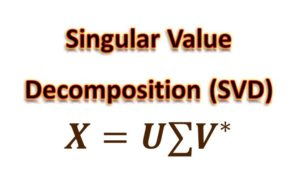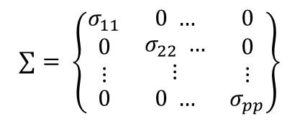# Singular Value Decomposition(SVD) – A Dimensionality Reduction Technique

Singular Value Decomposition (SVD) is a dimensionality reduction technique similar to PCA but more effective than PCA. It is considered as factorization of a data matrix into three matrices.Given a rectangular matrix A which is an n x p matrix, the SVD theorem shows that this matrix can  be represented as:

A = U∑V*

where

A is the original data matrix (n x p)

U is  the left singular vectors

∑  contains the singular values on its diagonal  as shown below

V* is right singular vectorsThis  singular values matrix is can be taught of as the eigen vectors  in the Principal Components Analysis. SVD can actually be interpreted in a similar way as PCA.

The first column of V corresponds to the largest singular value and the next column of V corresponds to the next largest singular value and so on.

This being the case, then if we multiply the singular values matrix with the original matrix, we would have:

XV = U∑V*V

Since V*V  = I, then we would have

XV = U∑

This means that we would have the scores(projection of the original data into the first principal component) after we have computed the SVD since we have U and ∑

T = U∑

This is really easy because since σ  is filled with zeroes,  all we need to do is to multiply every column of U by a single number (you can look at a Review of Matrix Operations).

So if we are interested in only two principal components, we take two columns of V

Tr = Urr

How Many Principal Components do we Pick

How then do we determine the number of principal components to pick? Whether 2 , 3, 4 or more.

Let’s first observe that the singular values (eigen values in PCA) are ordered. This means that they get smaller as we move down the diagonal. So we need to create a plot of the cumulative sum of the singular values from 1 to k against k.

The shape of this curve helps us to understand the data. From the plot we can see how many components needed to explain the variance in the data.

This would give us a plot like shown in Figure 1.0.

Since the singular values get smaller and smaller, the plot flattens out into a plateau as it gets to the maximum. Then we examine the shape of the curve. There are three options:

• It could have a sharp edge: In this case, the first few components gives us all the variance
• It could be a smooth curve: Here the variance depends on so many components
• It could be in between

When the curve reaches a plateau, then adding more components does not contribute any additional explanation to the data.

Another approach is to set some threshold on the percentage of the data that needs to be explained. In this case, when enough number of components have been added that explain the variance in the data up to this threshold, then we stop. For instance, how many singular values is needed to explain 90% of the data or 95% of the data and so on.#### kindsonthegenius

Kindson Munonye is currently completing his doctoral program in Software Engineering in Budapest University of Technology and Economics

View all posts by kindsonthegenius →

## One thought on “Singular Value Decomposition(SVD) – A Dimensionality Reduction Technique”

1.ปั้มไลค์ says:

Like!! Great article post.Really thank you! Really Cool.